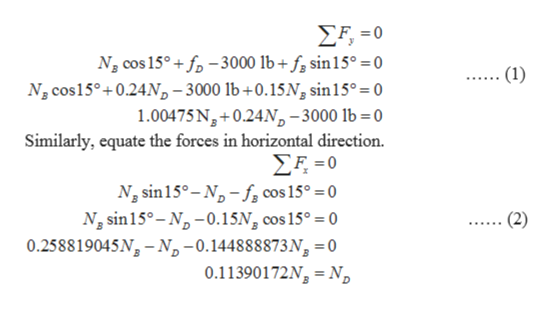Question
201 views

The coefficient of static friction between A and C and between B and D is μs = 0.24, and between A and B is μs′ = 0.15. Neglect the weight of each wedge. (Figure 1)

Determine the horizontal force P needed to pull out wedge A.
Express your answer to three significant figures and include the appropriate units.
check_circle

Step 1

Consider ND as the normal force by the wall D on block B, fD as the friction force due to surface B, fB as the friction force due to surface A, and NB as the normal force due to block A.

Draw the free body diagram of block B.

Step 2

Calculate the friction force fD.

Step 3

Equate the forces in ver...help_outlineImage TranscriptioncloseΣΕ-0 N cos 15°+ fp-3000 Ib+ f sin15° = 0 N2 cos15° 0.24N,-3000 Ib+0.15N, sin15° = 0 1.00475 Ng+0.24Np-3000 lb 0 Similarly, equate the forces in horizontal direction. ΣΕ-0 N sin 15°-N-fcos 15° = 0 Ng sin15°-N-0.15N, cos 15° = 0 0.258819045N-N-0.144888873N, 0 0.11390172Ng N (1) (2) fullscreen

### Want to see the full answer?

See Solution

#### Want to see this answer and more?

Solutions are written by subject experts who are available 24/7. Questions are typically answered within 1 hour.*

See Solution
*Response times may vary by subject and question.
Tagged in

### Mechanical Engineering